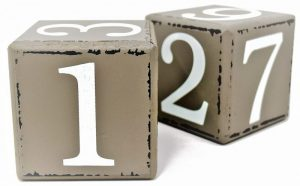Mathematics

# Real numbers

Real numbers are all those numbers that are included within rational numbers. They can be positive, negative and include the number zero, as in the case of irrational numbers. These numbers can be written in different ways, some of them very simple, generally used in simple mathematical operations, and in more complex forms. Also included in this group of numbers are whole number fractions that have in their denominator numbers that are not null.Related topics

## What are real numbers?

Real numbers are all those numbers found on the number line that make up the group of rational and irrational numbers, negative and positive, including the number zero.

## Which are the real numbers?

The system of real numbers is formed mainly by two large groups, the rational numbers, which are all those numbers that can be expressed as the division of two whole numbers, and the system of irrational numbers whose decimal representation is expansive, infinite and aperiodic. Rational numbers can also be divided into subgroups, among which we can mention: non whole fractions with their negative notations; whole numbers including negative numbers and positive integers; the latter in turn include natural numbers and zero.

## What are real numbers for?

Real numbers are digits that allow us to carry out all the mathematical operations necessary to solve a situation or problem. They also have as function, to designate the quantity of elements that have a certain group. They can be used to identify places or objects and to order and prioritize categories.

## Characteristics

Among the main characteristics of real numbers we can mention the following:

• They are formed by the union of rational and irrational
• They are a complete set.
• This type of numbers and the number line have a close relationship.
• For each real number there is a point that represents it within the number line.
• Natural numbers are complete, and it is an ordered
• They are numbers that have associativity and commutativity.
• They all have an order and are written consecutively.
• When they are used for counting, then we mean that they have a cardinal

## History

They appeared as a need for the people of the primitive era to tell and solve problems that were constantly presented to them. It is recorded that the Babylonians used them to count, for example, their animals. The Egyptians used the fractions for the first time through mathematicians such as Pythagoras. During the 19th century, two important mathematicians, Georg Cantor and Richard Dedekind, made constructions and systemizations of real numbers. These two historical figures in Mathematics managed to systematize real numbers using a series of advances invented by them.

## Classification

Real numbers are classified or divided into the following groups:

• Natural numbers: These are the numbers we normally use to count. They can start with 0 or with 1. They serve as a basis for making larger numbers, and they are numbers that have divisibility and number distribution. With them, you can add, subtract, multiply and divide.
• Integers: These are numbers that can be written without using a fraction. They are complete numbers and are used to express quantities, depths, temperatures. Together, they form the smallest group of real numbers.
• Rational numbers: These are the numbers that can be expressed as a fraction of two whole numbers, which have a numerator and a denominator. It is represented by the letter They can also be defined as types of equivalences of whole pairs.
• Irrational numbers: These are real numbers that are not rational numbers either. These numbers cannot be expressed as fractions. Among them we can mention the radius of a circumference, the golden number and the square root.

## Real numbers properties

The property of the real numbers can change depending on the type of operation, being this the case, then we have the following ones:

• Properties of the Sum
• Internal property: the result of adding two real numbers will result in another real number.
• Associative property: if there are more than two summands, it doesn’t matter which sum is done first, if the numbers are all real.
• Commutative property: the order of the digits will not alter the sum.
• Existence of the neutral element: any number that is added with 0 will result in the same number.
• Subtraction properties
• If the minuend or subtracting are positive, the subtraction will give a positive result, otherwise the result will be negative.
• If the minuend is negative and the subtracting positive, the addition is done, and the result has a minus sign.
• Subtracting a positive number is the same as adding a negative number.
• Subtracting a negative number is the same as adding a positive number.
• Properties of multiplication
• Internal
• Associative
• Commutative
• Distributive
• Inverse or opposite element.
• Common factor.

## Operations

The mathematical operations that can be performed using real numbers are: addition, multiplication, subtraction, division and potentiation or enhancement.

## Examples

• 120
• 1234512345…
• – ½
• √5
Written by Gabriela Briceño V.